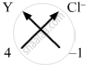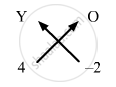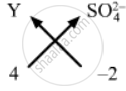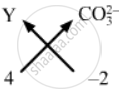# An Element Y Has a Valency of 4. Write the Formula for Its : Chloride Oxide Sulphate Carbonate Nitrate - Science

An element Y has a valency of 4. Write the formula for its :

1. chloride
2. oxide
3. sulphate
4. carbonate
5. nitrate

#### Solution

Formula for the listed compounds are as follows:

1. Crossing the valencies, chloride for the element Y will have the chemical formula YCl4.2. Crossing over the valencies, oxide for the element Y will have the chemical formula YO2.3. Crossing the valencies, sulphate for the element Y will have the chemical formula Y(SO4)2.4. Crossing the valencies, carbonate for the element Y will have the chemical formula Y(CO3)2.5. Crossing  the valencies, nitrate for the element Y will have the chemical formula Y(NO3)4.
Concept: Chemical Formula Or Molecular Formula
Is there an error in this question or solution?

#### APPEARS IN

Lakhmir Singh Class 9 Chemistry - Science Part 2
Chapter 3 Atoms and Molecules
Long Answers | Q 29.2 | Page 152